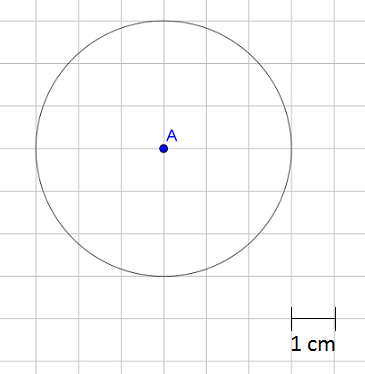# Geometry

## Objective

Determine the relationship between the area and radius of a circle and use it to solve problems.

## Common Core Standards

### Core Standards

?

• 7.G.B.4 — Know the formulas for the area and circumference of a circle and use them to solve problems; give an informal derivation of the relationship between the circumference and area of a circle.

## Criteria for Success

?

1. Approximate the area of a circle using a grid.
2. Informally derive the formula for the area of a circle using wedges of a circle.
3. Know the formula that relates the area and circumference of a circle: ${{A=\pi r^2}}$.
4. Use the formula ${{A=\pi r^2}}$ to solve problems.

## Tips for Teachers

?

Lessons 8 and 9 focus on the relationship between a circle’s area and its radius. In Lesson 8, students determine this relationship and the formula that represents it. In Lesson 9, students will solve real-world and mathematical problems involving area.

#### Fishtank Plus

• Problem Set
• Student Handout Editor
• Vocabulary Package

## Anchor Problems

?

### Problem 1

Use the centimeter grid to find an approximate area of the circle. Explain why your approximation is reasonable.### Problem 2

Use this applet from Academo, Area of a circle (Rearrangement Method) to informally derive the relationship between the area of a circle and its circumference

Start with a small number of sectors or wedges of the circle and then slowly increase the number. Click “rearrange” after each number. Ask:

• How are the circle wedges being rearranged?
• What shape are the rearranged pieces taking?
• What are the dimensions of the rearranged shape?
• How can you determine the area of a circle?

### Problem 3

Use the relationship between area and radius to solve the two problems below.

1. The radius of a circular patch of grass is 3 yards. What is the area of the patch of grass?
2. The area of a circular plate is approximately ${50.24 \space\mathrm{in^2}}$. What is the radius of the plate?

## Problem Set

?The following resources include problems and activities aligned to the objective of the lesson that can be used to create your own problem set.

• Include spiraled review from the unit, including procedural problems solving equations if additional practice is needed.

?

### Problem 1

A circle is divided into 16 equal wedges, as shown below. Explain or show how you can rearrange the pieces to determine the area of the circle.### Problem 2

Find the area of a circle that has a radius of 5 inches.

?